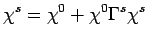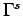Next: 3.4 Technical Aspects Up: 3.3 Approximations to the Previous: 3.3.2 Second Order in

## 3.3.3 Full QMC vertex

In the non-perturbative MSMB approach the full irreducible vertex, as obtained by the QMC, is considered. Within the QMC algorithm we are unable to determinedirectly and instead calculate the two-particle correlation function. The irreducible vertex function can be found by inverting the Bethe-Salpeter equation. In the spin-channel we have the two particle correlation function(3.25)

which is a matrix with elements both in frequency and momentum (the same holds for the charge channel). The irreducible spin vertex is denoted byandis the bare spin susceptibility respectively. The correlation functions are evaluated in the QMC by sampling over the configuration space (Hirsch-Hubbard-Stratonovich fields(2)) in one of two ways, each one posing its own challenges.

One possibility is directly evaluatingin frequency space. This requires the individual QMC Green's function to be Fourier transformed (FT) from the time domain. However, since the calculation is limited to finite time intervalsthe FT will incur substantial high-frequency artifacts. In theory this can be improved by means of a high-frequency conditioning, but no analytic form is available within the QMC calculation to facilitate such a conditioning. This resulting substantial artifacts are propagated into the irreducible vertex function.

Alternativelycan be evaluated in the time domain and only Fourier transformed once the QMC averaging is complete. This results in an accurate measurement of the two-particle correlation function by the QMC but is significantly more computationally expensive. Although this approach provides an accurate measure for, the Bethe-Salpeter equation can not be be used to solve forin the time domain and hence the QMC averagedhas to be Fourier transformed first. This once again results in similar problems associated with the FT. In the high temperature regime where higher order corrections inare not important a perturbative approach to high-frequency conditioning of the FT is very successful. However, as the temperature is lowered and the significance of higher order corrections within the vertex grows, this conditioning results in even larger artifacts. Therefore we use the frequency domain in our determination of.Next: 3.4 Technical Aspects Up: 3.3 Approximations to the Previous: 3.3.2 Second Order in
© Cyrill Slezak